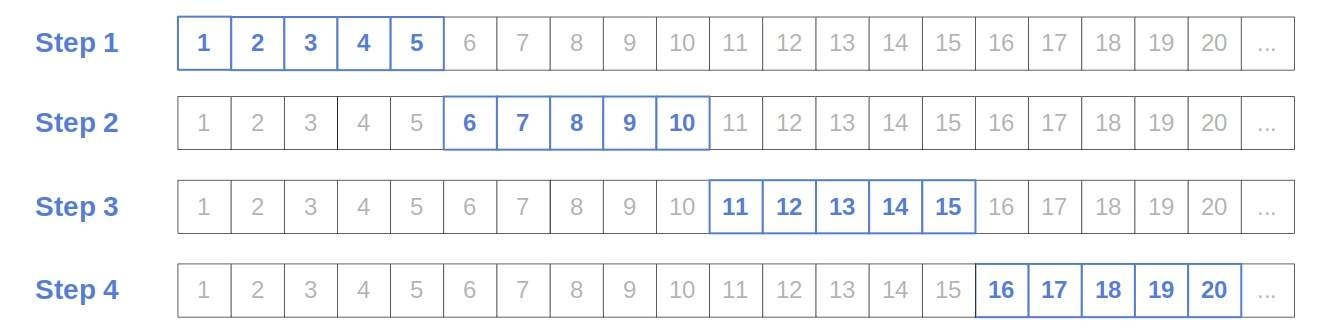## 1. Introduction

In this tutorial, we’ll present the Jump Search algorithm.

## 2. Search Problems

In a classical search problem, we have a valueand a sorted arraywithelements. Our task is to find the indexofin.

Ifis a linked list, it doesn’t have indices. Instead, each element is apair, so we’re looking for the item whose key is. We’ll denote theth element of the listasjust as we do with arrays.

However, ifis an array, we can access any element in antime (assuming thatfits into the main memory). In contrast, ifis a list, we need to followpointers to reach, so the complexity of access is. In the worst case, we want to reach the end of the list, so we traverse all theelements to get to it, which takestime.

That’s why an array search algorithm such as Binary Search isn’t suitable for singly-linked lists. It checks the elements ofback and forth, but going back isn’t anoperation whenis a list, and checkingafter() takes more than a constant time.

Jump Search is a list search algorithm but can handle arrays equally well. It assumes that its inputis a sorted linked list. That means that each element’s key should bethan the next one’s.

The algorithm partitionsinto the sub-lists of the same size,,,,and checks them iteratively until locating the one whose boundaries contain:Once the sub-list is found, the algorithm checks its elements one by one until findingor reaching the last one in the sub-list ifisn’t there.

The name comes from the jumps of sizebetween the steps. For instance, this is how the algorithm runs when:### 3.1. Pseudocode

Here’s the pseudocode:We can setto any value we like, but the algorithm’s complexity depends on our choice of.

### 3.2. Complexity Analysis

Let’s say that our list haselements. In the worst case, we check all thesub-lists’ boundaries and compare all the elements in the last sub-list to. So, we performcomparisons.

The minimum ofiswhen. So, if we setto, Jump Search will run intime with respect to the number of comparisons.

However, to reach the new sub-list’s end, we need to followpointers from the previous step’s. As a result, we traverse allnodes in the worst case. So, the algorithm has anworst-case time complexity if we consider node traversals. However, if a comparison is more complex than following a pointer, we can say that the complexity is.

### 3.3. Arrays

As we said before, Jump Search can handle arrays too. We prefer it over logarithmic Binary Search when going back (e.g., from testing ifto checking whether) is expensive or impossible.

That can happen whenis so large that it can’t fit into the working memory or is a stream of data we’re downloading from an external source. In the former case, requestingaftermight require loading new blocks from the disk into RAM. In the latter case, downloading individual elements is expensive since we get them over a network, so going back and forth like in Binary Search would be slow.

## 4. Conclusion

In this article, we presented the Jump Search algorithm. We use it to search singly-linked lists intime, but it can also search arrays.